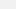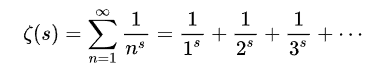A Mathematician May Have Just Solved a 160-Year-Old, \$1 Million Problem

This isn’t the first time Michael Atiyah has claimed to crack a big math problem and never followed up the claims with publication, however.

|
Sep 24 2018, 6:34pmAtiyah speaking at the Heidelberg Laureate Forum on September 24, 2018. Image: YouTube

A renowned mathematician has claimed to have developed a proof for the Riemann hypothesis, a 160-year-old math problem that carries a \$1 million bounty.

Michael Atiyah, a mathematician who has won several of the highest awards in mathematics, gave a lecture at Heidelberg Laureate Forum in Germany on Monday to explain his proof of the Riemann hypothesis, which was first posited by Bernhard Riemann in 1859 about numbers return a value of zero when used as an argument for a certain function—but he abandoned trying to provide a proof.

As Atiyah pointed out during his talk, Riemann’s hypothesis “has been verified numerically for millions and millions with every computer you can think of, but there is no proof.” Nevertheless, the hypothesis has had enormous practical value for mathematicians, who have used it to explain the strange distribution of prime numbers among other mathematical oddities.

If Atiyah’s proof is correct, it would be a big deal for the mathematics community—in the past 160 years furnishing a proof to the Riemann hypothesis has become one of the most vexing problems in mathematics. Since 2000 Clay Mathematics Institute has offered a \$1 million prize to the mathematician who publishes their results in a peer-reviewed journal and waits two years for other mathematicians to form possible objections to the solution. Although Atiyah demonstrated his proof on Monday, it hasn’t yet been accepted for publication in a peer-reviewed journal.

“The Riemann hypothesis has been proved, unless you’re the type of person who doesn’t believe in proof by contradiction,” Atiyah said during his Heidelberg lecture. “In that case, I have to go back and think again. But people usually accept proof by contradiction so I would argue my case that I deserve the prize.”

But many mathematicians expressed reservations about the legitimacy of the solution given that Atiyah has made claims about giving proofs for major math problems before, only to have his solutions fall apart under scrutiny or fail to be published.

If Atiyah’s proof doesn’t hold up, it wouldn’t be the first time a mathematician has claimed to have cracked Riemann’s hypothesis and failed. In 2015, an unknown Nigerian professor named Opeyemi Enoch claimed to have provided a proof for the Riemann hypothesis but the entire thing turned out to be a sham. Unlike Enoch, Atiyah has won both the Fields Medal and Abel Prize, which are basically like Nobels for mathematicians.

As Markus Pössel, a German astronomer who was present at Atiyah’s lecture, told me in an email, it’s too early to make a judgment on whether the proof is legitimate.

“Those who are specialists in this field do not yet have sufficient information to judge that claim properly,” Pössel said. “In particular Atiyah made use of an unusual function he called the ‘Todd function’ after one of his teachers. It’s not clear to me whether that function really exists in the form claimed by Atiyah. It’s certainly reasonable to be cautious about this.”

WHAT IS THE RIEMANN HYPOTHESIS?

In 1859, the mathematician Bernhard Riemann made a hypothesis about the when a particular function returns a value of zero. The hypothesis has a number of practical applications in mathematics, including as an explanation for the strange distribution of prime numbers, which are only divisible by themselves and one.

Riemann’s hypothesis is about values used in the zeta function, which produces either a convergent or divergent series of numbers depending on the value of s—known as the function’s argument—in the following sequence:Riemann zeta function. Image: Wikimedia Commons

Riemann’s insight was that the zeta function could also be extended to complex numbers, which are a combination of imaginary and real numbers. (Quick refresher: real numbers are those can be considered as points on a line, whereas imaginary numbers such as -1, which is represented as i, cannot. Thus, for example, 3 + 5 i is a complex number.)

As Edward Frenkel explained in a video for Numberphile, if you plug a real number into the zeta function, such as “2,” you’ll get the series “1+ ¼ + 1/9 + 1/16…” The more numbers that are discovered in this sequence and added together, the closer they approximate a certain total known as the limit. If the series approaches a limit, then it is considered a convergent series.

On the other hand, if a number like “-1” is used as the argument for the zeta function, it returns a series “1+2+3+4+5…” This type of series has no limit since the sum of the numbers keeps getting larger and is known as a divergent series.

Riemann argued that if a complex number is used as the argument for the zeta function, this results in a convergent series. When certain numbers are used as the real number input for a zeta function whose argument is a complex number, it returns a value of zero.

Some of these inputs are trivially easy to discover. For example, -2, -4, and -6 will all return zero. But what Riemann hypothesized was that if ½ was used as the real number for the complex argument of a zeta function, any imaginary number it paired with would also return zero. Thus ½ + 1 i, ½ + 2 i, ½ + 3 i, and so on would all return zero.

“For which values does the zeta function equal zero?” Frenkel said in the Numberphile video. “That is the million-dollar question.”

Atiyah’s proof claims to answer this question by relying on something he called the “Todd function,” named after the late mathematician and Atiyah’s former teacher J.A. Todd. As Pössel pointed out, the novelty of this function is the source of many mathematicians’ skepticism about Atiyah’s proof.

If Atiyah hopes to claim the \$1 million prize for cracking this millennium problem, the name given to the seven most difficult math problems according to the Clay Mathematics Institute, then the Todd function will have to stand up to rigorous scrutiny by other mathematicians over the next two years.

So far, only one of the seven millennium problems has been solved, although dozens of solutions to the various problems have been proposed. This speaks to the difficulty of the problems at hand and also to the importance of peer review in mathematics. Even if Atiyah’s proof ultimately misses the mark, his solution is sure to be at the forefront of some of the world’s most brilliant mathematicians for the next few years.